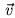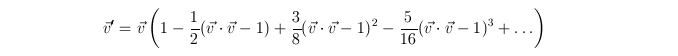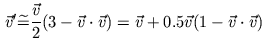Next: Tangent Space Up: Parameter Interpolation and Normalization Previous: Cube-Map

### Register Combiners

A faster technique for normalization without using texture maps is to use the register combiners extension . With register combiners only addition, subtraction, multiplication and dot products are possible (Section 3.3). Direct computation of the normalization equation shown in Formula 4 is not possible. Given that the vectoris derived from the interpolation of a unit-length vector across the polygon and the angle between the per-vertex vectors is not too big, this expression can be approximated by a Taylor series (Formula 5).(5)

Given the assumptions mentioned above and the limited 8 bit precision in the register combiners this expressions is truncated after the linear term (Formula 6).(6)

The last expression can be implemented directly with register combiner arithmetic in two general combiner stages.

Gerald Schröcker 2002-03-21